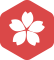### 在Jbuilder7中TableDataSet中的数据在JdbTable中显示的精度问题。在线等候...

Java > Java SE [问题点数：100分，结帖人namowen]spczhou

TensorflowAPI------tf.data.Dataset使用

Tensorflow API: tf.data.Dataset使用 Tensorflow之前主要用的数据读取方式主要有： 建立placeholder，然后使用feed_dict将数据feed进placeholder进行使用。使用这种方法十分灵活，可以一下子将所有数据读入...

tensorflow使用tf.data.Dataset 处理大型数据

tf.data.Dataset图像预处理详解

Tensorflow 数据读取 tf.data.Dataset API 相关介绍

TensorFlow数据读取机制：文件队列 tf.train.slice_input_producer和 tf.data.Dataset机制

TensorFlow数据读取机制：文件队列 tf.train.slice_input_producer和tf.data.Dataset机制 之前写了一篇博客，关于《Tensorflow生成自己的图片数据集TFrecord》，项目做多了，你会发现将数据转为TFrecord格式，实在...

e.target.dataset与e.currentTarget.dataset获取不到定义的值

uniapp:使用data-名称为函数传参，并e.currentTarget.dataset.名称获取

tf.data.Dataset读取数据的几种方式案例

tensorflow2 简单的数据加载5步法

How to use tf.data.Dataset API（读取数据

tensorflow使用tf.keras.Mode写模型并使用tf.data.Dataset作为数据输入

【网上开源的数据集】交通 数据集：【数据集-交通标志 红绿灯】红绿灯 交通标志

tf.data.Dataset.from_tensor_slices( )

Pytorchdataset类——创建适应任意模型的数据集接口

Tensorflow.Dataset中map，shuffle，repeat，batch的总结

Dataset API是TensorFlow 1.3版本引入的一个新的模块，主要服务于数据读取，构建输入数据的pipeline。 Google官方给出的Dataset API的类图： 我们本文只关注Dataset的一类特殊的操作：Transformation，即map，...

tf.data.Dataset的一些小坑

dataset = dataset.batch(batch_size).repeat(epochs) 用来说明我们需要对整个数据集进行多少个epochs,每次的输入大小是多少个batch. 注意: 如果我们的数据集的数量为N,而N%batch_size刚好能整除的话,上述代码是...

Dataset.Tables.Select从一个Dataset提取符合要求的数据

DataSet ds1= new DataSet(); DataTable dt1 = new DataTable(); dt1 = ds2.Tables.Clone();//复制ds2..Tables表的架构 ds2.Tables.Select("答卷IP like '" + XmlstructCountry.strName + "*'")....

TensorFlow全新的数据读取方式：Dataset API——tf.data.Dataset

tf.dataset 使用 python generator 加载和预处理数据dataset.map 对数据进行额外加工

tf.graph部分 使用 dataset.map 对输入数据进行加工 import tensorflow as tf # import tensorlayer as tl import numpy as np # 生成数据集 # x_train, y_train, x_test, y_test = tl.fil...

tensorflow tf.data.Dataset.from_generator方法详解

shapes = (tf.TensorShape([None, None]), tf.TensorShape([10, 10])) ...# dataSet output_types参数必选，output_shapes参数可选，不选会直接适配数据的shape # 参数就是一个元组 data_set = tf.data...

【微信小程序】e.target.id和e.currentTarget.dataset.id的区别

180512 tensorflow数据集tf.data.Dataset的基本操作

import tensorflow as tf import numpy as np import matplotlib.pyplot as plt ...# Step-1： 数据集实例化 dataset = tf.data.Dataset.from_tensor_slices(your real data) input_data = np.arange...

tf.data.Dataset.from_tensor_slices的用法

tf.data.Dataset.from_tensor_slices 该函数是dataset核心函数之一，它的作用是把给定的元组、列表和张量等数据进行特征切片。切片的范围是从最外层维度开始的。如果有多个特征进行组合，那么一次切片是把每个组合的...

Tensorflow2.0学习（八） — tf.dataset自定义图像数据